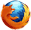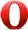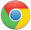# JavaScript floor() 方法JavaScript Math 对象

Math.floor(1.6);

1

## 定义和用法

floor() 方法返回小于等于x的最大整数。

## 浏览器支持Math.floor(x)

x 必需。任意数值或表达式。

## 返回值

Number 小于等于 x，且与 x 最接近的整数。

## 技术细节

 JavaScript 版本： 1

## 实例

var a=Math.floor(0.60);
var b=Math.floor(0.40);
var c=Math.floor(5);
var d=Math.floor(5.1);
var e=Math.floor(-5.1);
var f=Math.floor(-5.9);

a,b,c,d,e, 和 f 输出结果:

0
0
5
5
-6
-6JavaScript Math 对象